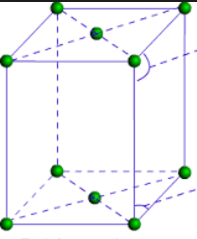#### Structure of a mixed oxide is cubic close - packed (c.c.p). The cubic unit cell of mixed oxide is composed of oxide ions. One fourth of the tetrahedral voids are occupied by divalent metal A and the octahedral voids are occupied by a monovalent metal B. The formula of the oxide is: Option 1) A2BO2 Option 2) A2B3O4 Option 3) AB2O2 Option 4) AB O2As we learnt in

No. of atoms(z) for end centered unit cell -

Lattice point: at corners and one pair of opposite face centers.

For  end centered cubic, z=2

- whereinGiven that its a c.c.p and unit cell is composed of oxide ions.

So,Octahedral voidsB = 4

Summing up, the formula isOption 1)

A2BO2

Incorrect option

Option 2)

A2B3O4

Incorrect option

Option 3)

AB2O2

Correct option

Option 4)

AB O2

Incorrect option